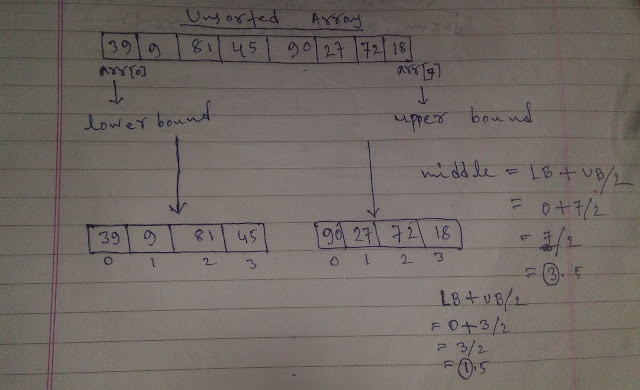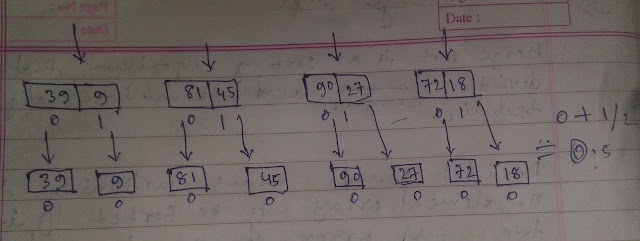# Merge Sort - CodeTextPro

## Merge sort is a sorting algorithm that uses divide, conquer and combine algorithm technic.

Divide: Divide means partitioning the n- element array to be sorted into two sub-array of n/2 elements. If A is an array containing 0 or 1 element, then it's already sorted. However, if there are more elements in the array divide A* two sub-array. Each containing about half of the elements of A.

Conquer: Conquer means sorting the two sub-array recurshiply using merge sort.

Combine: Combine means merging the two sorted sub-array of size n/2 to produce the sorted array of 'n' elements.Merge SortMerge Sort
Divide and Conquer the array.Merge Sort
Combine the elements from a sorted array.